Coming to Statistica
in 2022/2023

Zoom tutorials
using Explaineverything app
We a record sessions for you to listen to later

CALL STATISTICA NOW

.

E-mail     anne@statistica.com.au

# Place         Willetton, Western Australia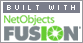# Mathematics & Statistic Tutor Perth - SPSS Help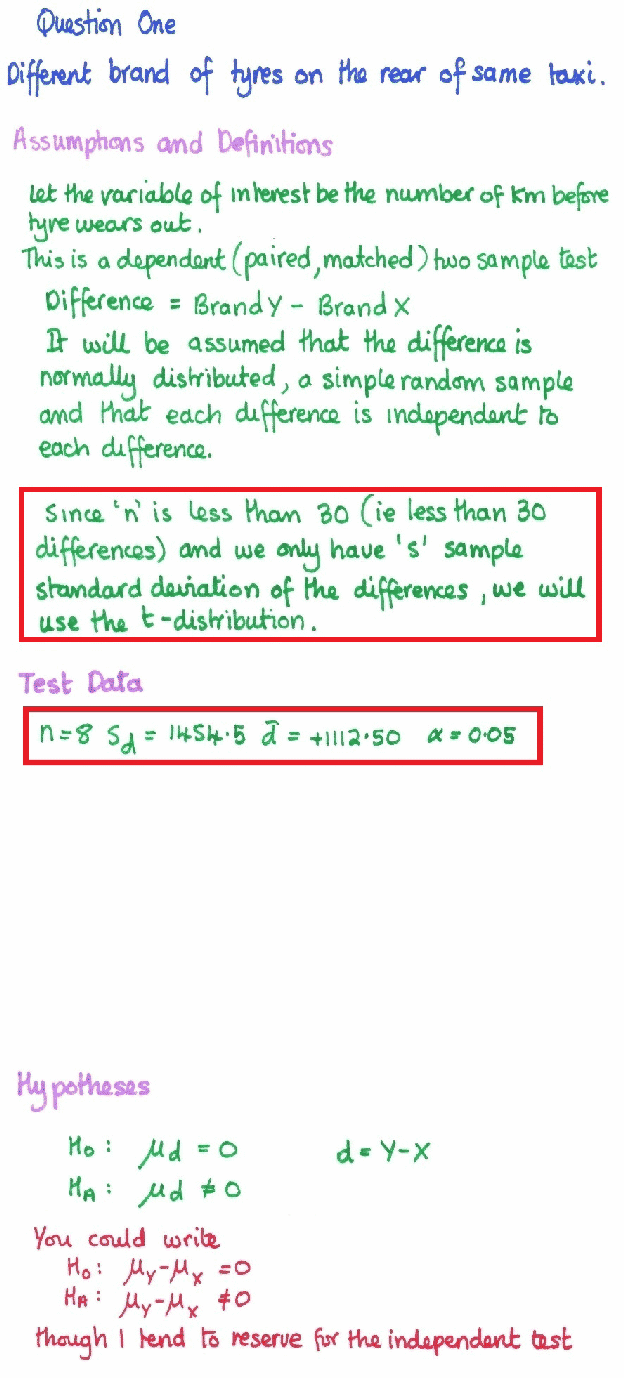Tests of Normality Kolmogorov-Smirnova Shapiro-Wilk Statistic df Sig. Statistic df Sig. difference .172 8 .200* .956 8 .769 *. This is a lower bound of the true significance. a. Lilliefors Significance Correction

 Descriptives Statistic Std. Error difference Mean 1112.5000 514.23921 95% Confidence Interval for Mean Lower Bound -103.4825 Upper Bound 2328.4825 5% Trimmed Mean 1086.1111 Median 1150.0000 Variance 2115535.714 Std. Deviation 1454.48813 Minimum -900.00 Maximum 3600.00 Range 4500.00 Interquartile Range 2325.00 Skewness .241 .752 Kurtosis .102 1.481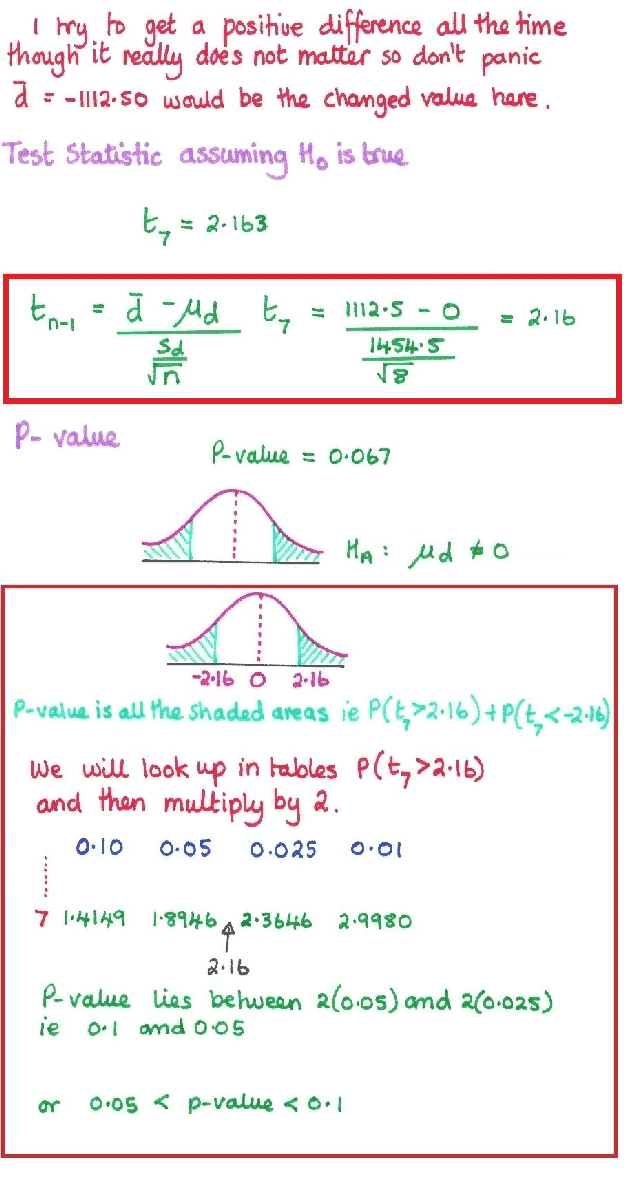Paired Samples Test Paired Differences t df Sig. (2-tailed) Mean Std. Deviation Std. Error Mean 95% Confidence Interval of the Difference Lower Upper Pair 1 Y - X 1112.50000 1454.48813 514.23921 -103.48251 2328.48251 2.163 7 .067
 Paired Samples Test Paired Differences t df Sig. (2-tailed) Mean Std. Deviation Std. Error Mean 95% Confidence Interval of the Difference Lower Upper Pair 1 Y - X 1112.50000 1454.48813 514.23921 -103.48251 2328.48251 2.163 7 .067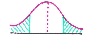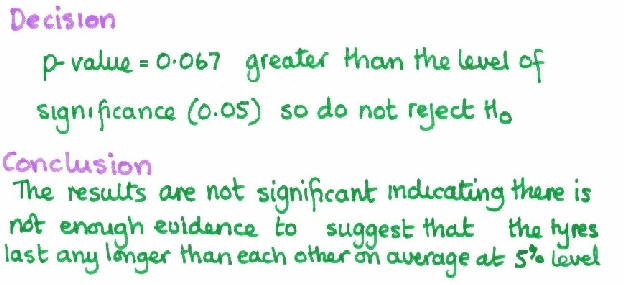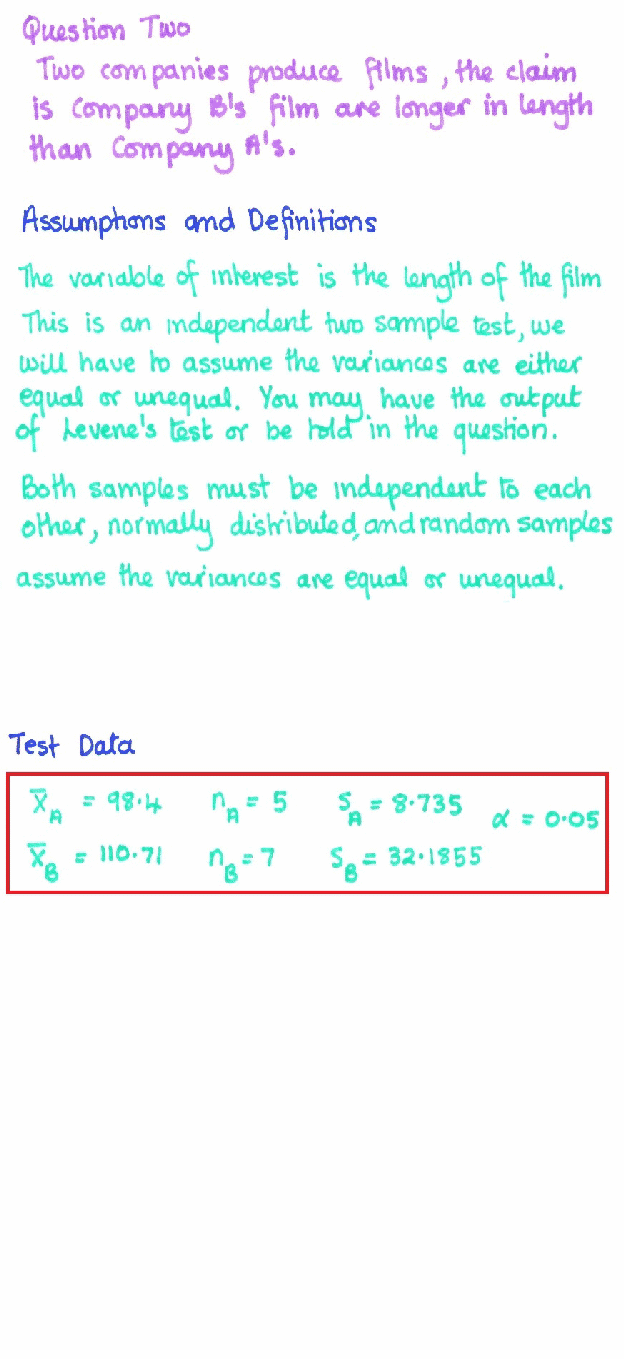Tests of Normalitya Kolmogorov-Smirnovb Shapiro-Wilk Statistic df Sig. Statistic df Sig. RunTime .118 5 .200* .999 5 .999 *. This is a lower bound of the true significance. a. Company = A
 Tests of Normalitya Kolmogorov-Smirnovb Shapiro-Wilk Statistic df Sig. Statistic df Sig. RunTime .236 7 .200* .839 7 .098 *. This is a lower bound of the true significance. a. Company = B

 Descriptives Company Statistic Std. Error RunTime A Mean 98.4000 3.90640 95% Confidence Interval for Mean Lower Bound 87.5541 Upper Bound 109.2459 5% Trimmed Mean 98.3889 Median 98.0000 Variance 76.300 Std. Deviation 8.73499 Minimum 87.00 Maximum 110.00 Range 23.00 Interquartile Range 16.00 Skewness .057 .913 Kurtosis -.309 2.000 B Mean 110.7143 12.16497 95% Confidence Interval for Mean Lower Bound 80.9477 Upper Bound 140.4809 5% Trimmed Mean 108.7381 Median 97.0000 Variance 1035.905 Std. Deviation 32.18547 Minimum 82.00 Maximum 175.00 Range 93.00 Interquartile Range 35.00 Skewness 1.563 .794 Kurtosis 2.518 1.587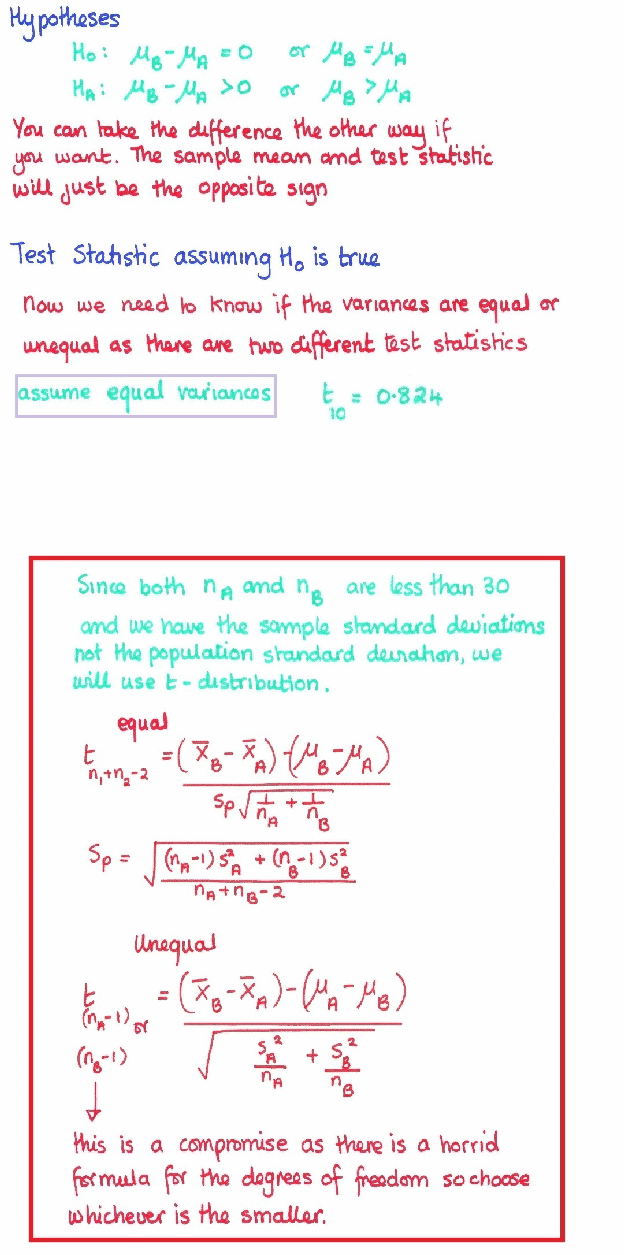Independent Samples Test Levene's Test for Equality of Variances t-test for Equality of Means F Sig. t df Sig. (2-tailed) Mean Difference Std. Error Difference 95% Confidence Interval of the Difference Lower Upper RunTime Equal variances assumed 3.887 .077 .824 10 .429 12.31429 14.95207 -21.00100 45.62957 Equal variances not assumed .964 7.187 .366 12.31429 12.77679 -17.73974 42.36831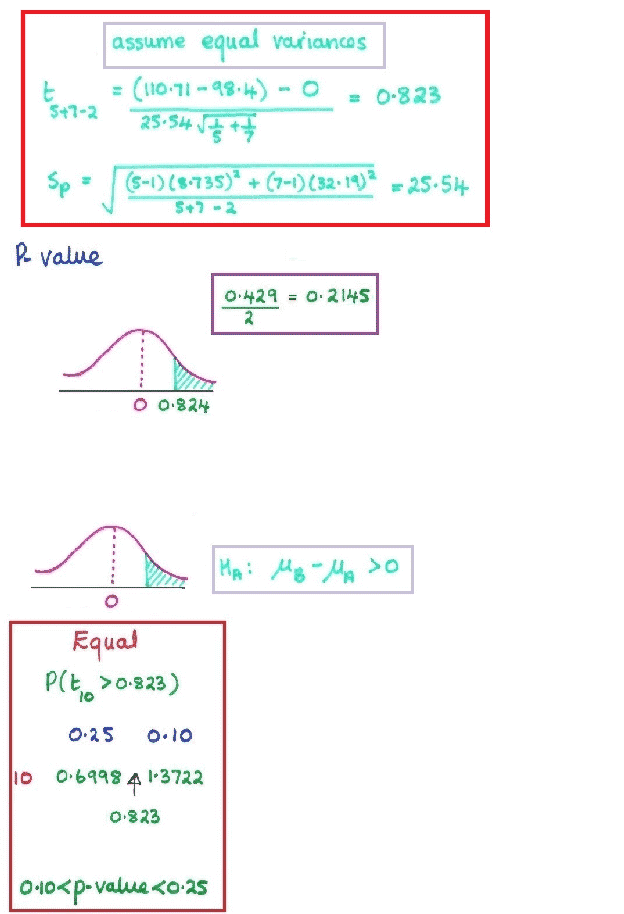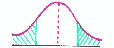Video Explanation Link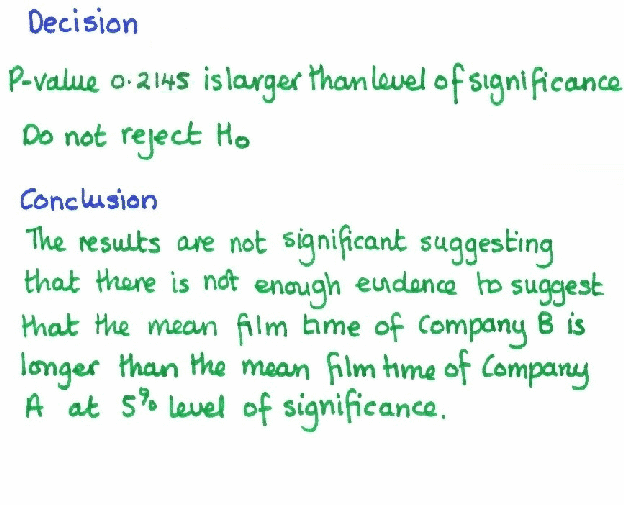[Home] [Parents] [Contact us] [Services] [Mathematics] [Statistics] [School]